## ML Aggarwal Class 7 Solutions for ICSE Maths Chapter 4 Exponents and Powers Check Your Progress

Question 1.
Find the value of each of the following:
(i) (-3)3 × 52
(ii) (-1)501 × [(27)4 ÷ (9)5]
(iii) $$\left( -3\frac { 1 }{ 2 } \right) ^{ 3 }$$
Solution: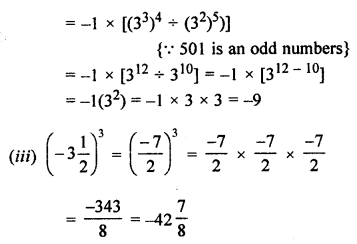Question 2.
Simplify the following: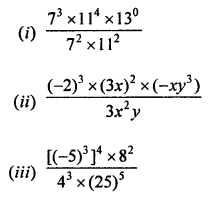Solution: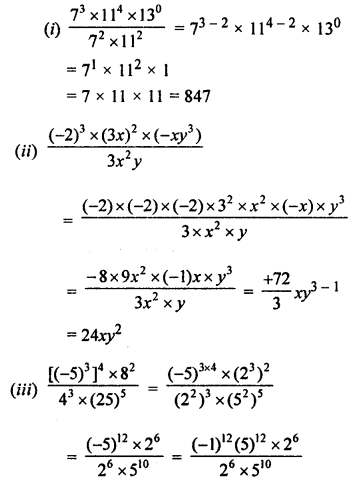Question 3.
Simplify and write the following in exponential form:Solution:Question 4.Solution:Question 5.
Write the prime factorisation of the following numbers in the exponential form:
(i) 24000
(ii) 12600
(iii) 14157
Solution:
(i) 24000= 2 × 2 × 2 × 2 × 2 × 2 × 3 × 5 × 5 × 5
= 26 × 31 × 53
(ii) 12600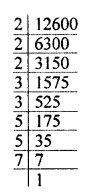= 2 × 2 × 2 × 3 × 3 × 5 × 5 × 7
= 23 × 32 × 52 × 71
(iii) 14157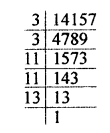= 3 × 3 × 11 × 11 × 13
= 32 × 112 × 131

Question 6.
Express the numbers appearing in the following statements in scientific notation:
(i) The earth has 1,353,000,000 cubic km of water.
(ii) The population of India was about 1,027,000,000 in march, 2001.
(iii) 60,230,000,000,000,000,000,000 molecules are contained in a drop of water.
Solution:
(i) The earth has 1,353,000,000 cubic km of water.
= 1.353 × 109 cubic km
(ii) The population of India was about 1,027,000,000 in march, 2001.
= 1.027 × 109
(iii) 60,230,000,000,000,000,000,000 molecules are contained in a drop of water.
6.023 × 1022 molecules

Question 7.
Compare the following numbers:
(i) 5.976 × 1024; 8.689 × 1023
(ii) 3.7662 × 1017; 3.7671 × 1017
Solution:
(i) 5.976 × 1024; 8.689 × 1023
Here in 5.976 × 1024, 102 is greater then in 8.689 × 1023
5.976 × 1024 > 8.689 × 1023
(ii) 3.7662 × 1017; 3.7671 × 1017
Here 1017 is multiplied in both
and 76671 > 7662
3.76671 × 1017 > 3.7662 × 1017